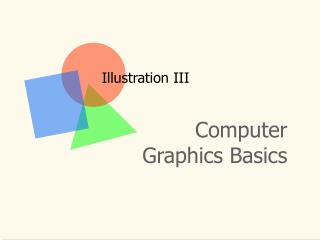DownloadDownload PresentationIllustration III

Illustration III

Télécharger la présentationIllustration III

- - - - - - - - - - - - - - - - - - - - - - - - - - - E N D - - - - - - - - - - - - - - - - - - - - - - - - - - -
Presentation Transcript

1. Illustration III Computer Graphics Basics

2. Q:What is a model? • A useful representation • It contains significant elements of the object being modeled, but not all elements • It is useful • Example: A road map

3. Q:What is a model? • In animation, a mathematical description of the object • Types of modeling • Surface • Solid • Particle-systems

4. Surface modeling • Only surface of object is represented • Model is hollow • Often uses either polygonal mesh or spline patches

5. Solid modeling • Both surface and interior attributes are represented • Model is solid • Allows for Boolean combinations of models

6. Particle systems • Comprised of particles • Models do not have a fixed or stable shape • Examples: Smoke, rain, fire, clouds

7. Polygonal Models • Point • 0-dimensional • Literally, a point in some n-space • Line • 1-dimensional (length) • Two points define a line • Plane • 2-dimensional (length, width) • Three points define a plane

8. Polygonal Models • Edge or Line segment • A portion of a line that falls between two points on the line • Polygon • A closed transit through a series of points • Surface • A polygonal section of a plane, where all points are in the plane

9. Polygonal Models • Polygon count • Number of polygons in a model • Polygon reduction • Aka polygon thinning or polygon culling • Eliminate/merge polygons to reduce polygon count • Polygon Expansion • Adds/divides polygons to increase count

10. Polygonal Models • Polygon Rounding • Adds/divides polygons to smoothorround sharp angles

11. Polygonal Models • Mesh • Polygons organized into rows and columns • Topology • Description of surface organization

12. Splines & Patches • Linear Approximation (Polyline) • A series of connected line segments • As the number of segments increases, so does the resolution of the polyline • Be careful! This may result in too much data!

13. Splines & Patches • Spline • Parameterized curve defines by a collection of control points and cubic equations • Interpolated spline • Control points on curve • Cardinal spline • Endpoints not on curve • Approximated spline • Control points not all on curve

14. Splines & Patches • Patch • Surface defined by cubic spline curves in two directions • Bicubic

15. Y+ Axes Origin X+ Z+ Coordinate Systems

16. Coordinate Systems • Right-handed • Z+ axis points towards viewer • Left-handed • Z+ axis points away from viewer

17. Views • Perspective • Things farther away look smallerThings closer look larger • Orthographic • Same size regardless of distance from viewer • Top, Bottom, Front, Back, Left and Right views

18. Geometric Primitives Sphere Cube Cylinder Torus

19. Transformations • Scale • Resize with respect to a given axis • Rotate • Spin with respect to a given axis • Translate • Move to a new position